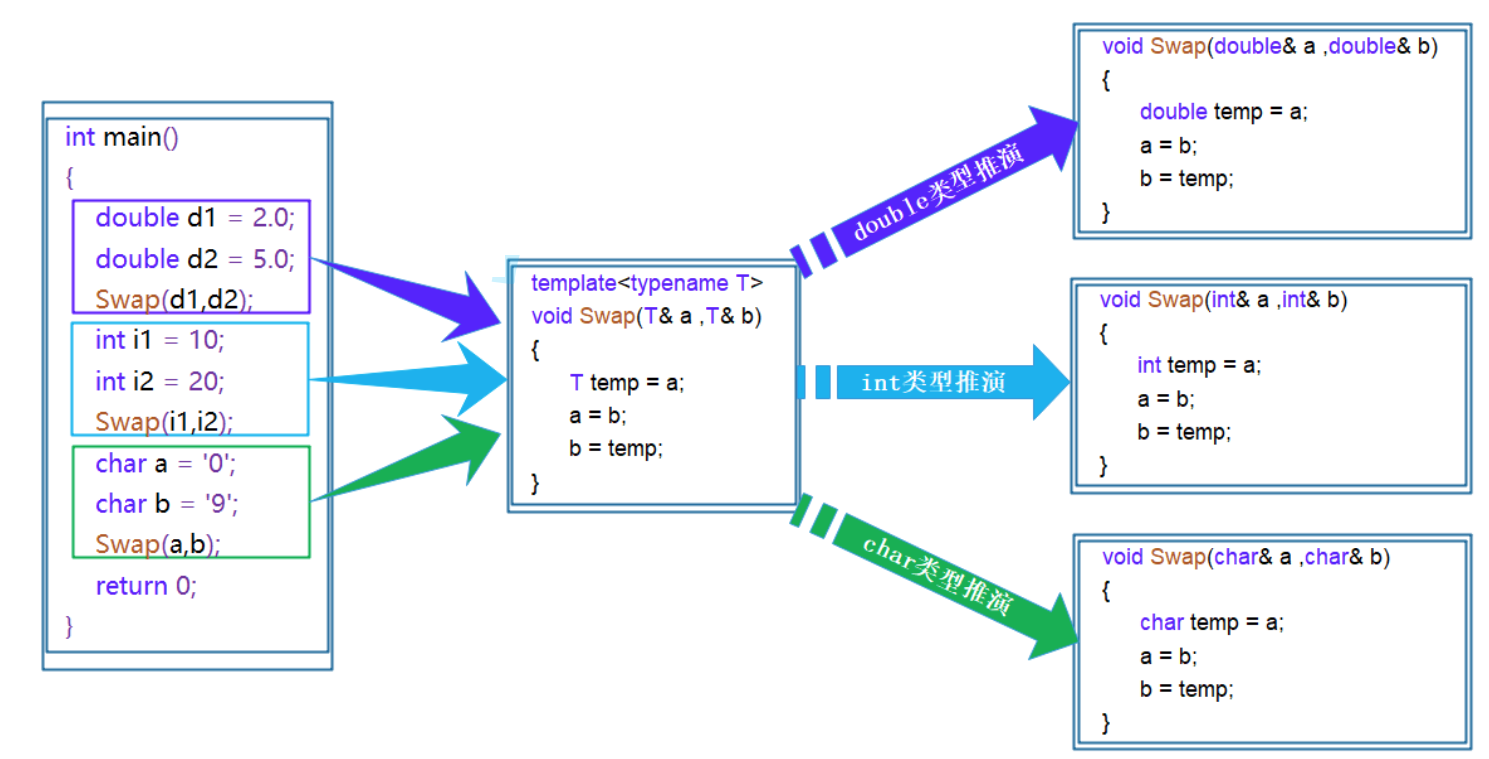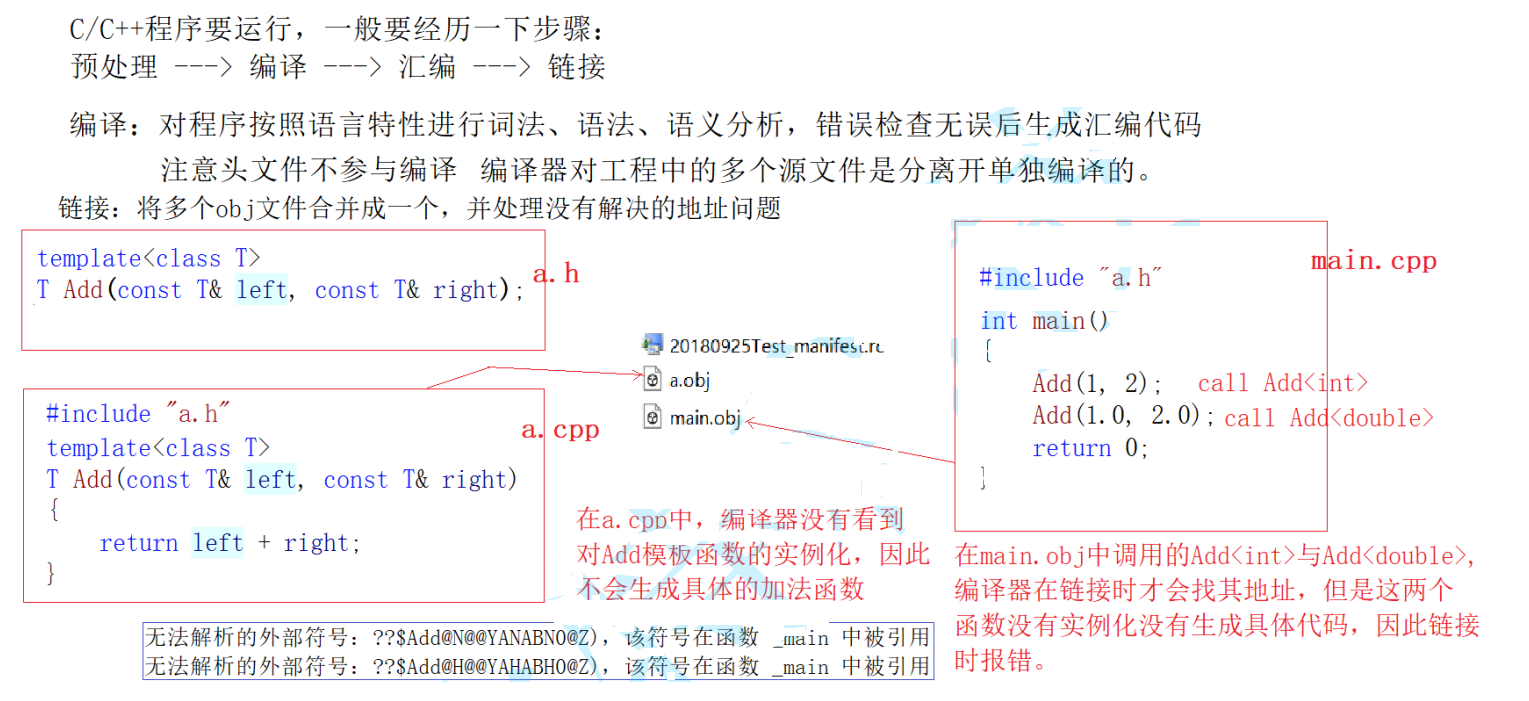• 累计撰写 270 篇文章
• 累计创建 6 个标签
• 累计收到 1 条评论

### 目 录CONTENT# C++中的模版.md2021-08-22 / 0 评论 / 0 点赞 / 15 阅读 / 7,126 字 / 正在检测是否收录...

# C++中的模版

@[toc]

## 一：范型编程

•  1.什么是范型编程？

• 泛型编程：编写与类型无关的通用代码，是代码复用的一种手段。模板是泛型编程的基础

•  如何实现一个通用的交换函数呢？

void Swap(int& left, int& right)
{
int temp = left;
left = right;
right = temp;
}

void Swap(double& left, double& right)
{
double temp = left;
left = right;
right = temp;
}

void Swap(char& left, char& right)
{
char temp = left;
left = right;
right = temp;
}

......


1. 重载的函数仅仅只是类型不同，代码的复用率比较低，只要有新类型出现时，就需要增加对应的函数
2. 代码的可维护性比较低，一个出错可能所有的重载均出错

•  3.模版的分类：
• 函数模版
• 类模版

## 二、函数模板

•  函数模板概念
• 函数模板代表了一个函数家族，该函数模板与类型无关，在使用时被参数化，根据实参类型产生函数的特定类型版本
•  函数模板格式
template<typename T1, typename T2,......,typename Tn>


template<typename T>
void Swap( T& left, T& right)
{
T temp = left;
left = right;
right = temp;
}

•  注意：typename是用来定义模板参数关键字，也可以使用class(切记：不能使用struct代替class)

•  函数模板的原理

• 模板是一个蓝图，它本身并不是函数，是编译器用使用方式产生特定具体类型函数的模具。所以其实模板就是将本来应该我们做的重复的事情交给了编译器• 在编译器编译阶段，对于模板函数的使用，编译器需要根据传入的实参类型来推演生成对应类型的函数以供调用。
• 比如：当用double类型使用函数模板时，编译器通过对实参类型的推演，将T确定为double类型，然后产生一份专门处理double类型的代码，对于字符类型也是如此。
•  函数模板的实例化
• 用不同类型的参数使用函数模板时，称为函数模板的实例化。模板参数实例化分为：隐式实例化和显式实例化。
• 隐式实例化：让编译器根据实参推演模板参数的实际类型，一般不进行类型转化
template<class T>
T Add(const T& left, const T& right)
{
return left + right;
}

int main()
{
int a1 = 10, a2 = 20;
double d1 = 10.0, d2 = 20.0;
/*

*/
// 此时有两种处理方式：1. 用户自己来强制转化 2. 使用显式实例化
return 0;
}

• 显式实例化：在函数名后的<>中指定模板参数的实际类型，可能会发生类型转化
int main(void)
{
int a = 10;
double b = 20.0;
// 显式实例化
return 0;
}

• 如果类型不匹配，编译器会尝试进行隐式类型转换，如果无法转换成功编译器将会报错
•  模板参数的匹配原则
• 一个非模板函数可以和一个同名的函数模板同时存在，而且该函数模板还可以被实例化为这个非模板函数
// 专门处理int的加法函数
{
return left + right;
}
// 通用加法函数
template<class T>
{
return left + right;
}
void Test()
{
}

• 对于非模板函数和同名函数模板，如果其他条件都相同，在调动时会优先调用非模板函数而不会从该模板产生出一个实例。
• 如果模板可以产生一个具有更好匹配的函数， 那么将选择模板
// 专门处理int的加法函数
{
return left + right;
}
// 通用加法函数
template<class T1, class T2>
{
return left + right;
}
void Test()
{
}


## 三、类模版

•  类模板的定义格式
template<class T1, class T2, ..., class Tn>
class 类模板名
{
// 类内成员定义
};

// 动态顺序表
// 注意：Vector不是具体的类，是编译器根据被实例化的类型生成具体类的模具
template<class T>
class Vector
{
public :
Vector(size_t capacity = 10)
: _pData(new T[capacity])
, _size(0)
, _capacity(capacity)
{}
// 使用析构函数演示：在类中声明，在类外定义。
~Vector();
void PushBack(const T& data)；
void PopBack()；
// ...
size_t Size() {return _size;}
T& operator[](size_t pos)
{
assert(pos < _size);
return _pData[pos];
}
private:
T* _pData;
size_t _size;
size_t _capacity;
};

// 注意：类模板中函数放在类外进行定义时，需要加模板参数列表
template <class T>
Vector<T>::~Vector()
{
if(_pData)
delete[] _pData;
_size = _capacity = 0;
}

•  类模版的实例化
• 类模板实例化与函数模板实例化不同，类模板实例化需要在类模板名字后跟<>，然后将实例化的类型放在<>中即可，类模板名字不是真正的类，而实例化的结果才是真正的类
// Vector类名，Vector<int>才是类型
Vector<int> s1;
Vector<double> s2


## 四、非类型模板参数

•  1.模板参数分类类型形参与非类型形参
• 类型形参即：出现在模板参数列表中，跟在class或者typename之类的参数类型名称。
• 非类型形参，就是用一个常量作为类(函数)模板的一个参数，在类(函数)模板中可将该参数当成常量来使用
namespace wolf
{
// 定义一个模板类型的静态数组
template<class T, size_t N = 10>
class array
{
public:
T& operator[](size_t index){return _array[index];}
const T& operator[](size_t index)const{return _array[index];}
size_t size()const{return _size;}
bool empty()const{return 0 == _size;}
private:
T _array[N];
size_t _size;
}；
}


• 浮点数、类对象以及字符串是不允许作为非类型模板参数的。
• 非类型的模板参数必须在编译期就能确认结果
•  2 . 模板的特化
• 概念：通常情况下，使用模板可以实现一些与类型无关的代码，但对于一些特殊类型的可能会得到一些错误的结果，
template<class T>
bool IsEqual(T& left, T& right)
{
return left == right;
}
void Test()
{
char* p1 = "hello";
char* p2 = "world";
if(IsEqual(p1, p2))
cout<<p1<<endl;
else
cout<<p2<<endl;
}

•  函数模板的特化步骤：
1. 必须要先有一个基础的函数模板
2. 关键字template后面接一对空的尖括号<>
3. 函数名后跟一对尖括号，尖括号中指定需要特化的类型
4. 函数形参表: 必须要和模板函数的基础参数类型完全相同，如果不同编译器可能会报错误。
template<>
bool IsEqual<char*>(char*& left, char*& right)
{
if(strcmp(left, right) > 0)
return true;
return false;
}

•  类模板特化
1. 全特化
全特化即是将模板参数类表中所有的参数都确定化
template<class T1, class T2>
class Data
{
public:
Data() {cout<<"Data<T1, T2>" <<endl;}
private:
T1 _d1;
T2 _d2;
};

template<>
class Data<int, char>
{
public:
Data() {cout<<"Data<int, char>" <<endl;}
private:
int _d1;
char _d2;
};

1. 偏特化
任何针对模版参数进一步进行条件限制设计的特化版本。比如对于以下模板类：
template<class T1, class T2>
class Data
{
public:
Data() {cout<<"Data<T1, T2>" <<endl;}
private:
T1 _d1;
T2 _d2;
};


• 部分特化
// 将第二个参数特化为int
template <class T1>
class Data<T1, int>
{
public:
Data() {cout<<"Data<T1, int>" <<endl;}
private:
T1 _d1;
int _d2;
};

• 参数更进一步的限制
偏特化并不仅仅是指特化部分参数，而是针对模板参数更进一步的条件限制所设计出来的一个特化版本
//两个参数偏特化为指针类型
template <typename T1, typename T2>
class Data <T1*, T2*>
{
public:
Data() {cout<<"Data<T1*, T2*>" <<endl;}
private:
T1 _d1;
T2 _d2;
};

//两个参数偏特化为引用类型
template <typename T1, typename T2>
class Data <T1&, T2&>
{
public:
Data(const T1& d1, const T2& d2)
: _d1(d1)
, _d2(d2)
{
cout<<"Data<T1&, T2&>" <<endl;
}
private:
const T1 & _d1;
const T2 & _d2;
};

void test2 ()
{
Data<double , int> d1; // 调用特化的int版本
Data<int , double> d2; // 调用基础的模板
Data<int *, int*> d3; // 调用特化的指针版本
Data<int&, int&> d4(1, 2); // 调用特化的指针版本
}

•  模版特化的应用（类型萃取）

//为了将内置类型与自定义类型区分开，给出以下两个类分别代表内置类型与自定义类型
// 代表内置类型
struct TrueType
{
static bool Get(){return true;}
};

// 代表自定义类型
struct FalseType
{
static bool Get(){return false;}
};

//给出以下类模板，将来用户可以按照任意类型实例化该类模板
template<class T>
struct TypeTraits
{ typedef FalseType IsPODType; };

//对上述的类模板进行以下方式的实例化
template<>
struct TypeTraits<char>
{ typedef TrueType IsPODType;};

template<>
struct TypeTraits<short>
{ typedef TrueType IsPODType;};

template<>
struct TypeTraits<int>
{ typedef TrueType IsPODType; };

template<>
struct TypeTraits<long>
{ typedef TrueType IsPODType; };
// ... 所有内置类型都特化一下

//通过对TypeTraits类模板重写改写方式四中的Copy函数模板，来确认所拷贝对象的实际类型
/*
T为int：TypeTraits<int>已经特化过，程序运行时就会使用已经特化过的TypeTraits<int>, 该类中的
IsPODType刚好为类TrueType，而TrueType中Get函数返回true，内置类型使用memcpy方式拷贝
T为string：TypeTraits<string>没有特化过，程序运行时使用TypeTraits类模板, 该类模板中的IsPODType

*/
template<class T>
void Copy(T* dst, const T* src, size_t size)
{
if(TypeTraits<T>::IsPODType::Get())
memcpy(dst, src, sizeof(T)*size);
else
{
for(size_t i = 0; i < size; ++i)
dst[i] = src[i];
}
}

int main()
{
int a1[] = {1,2,3,4,5,6,7,8,9,0};
int a2;
Copy(a2, a1, 10);
string s1[] = {"1111", "2222", "3333", "4444"};
string s2;
Copy(s2, s1, 4);
return 0;
}

•  4.模版的分离编译
•  1.什么是分离编译？
• 一个程序（项目）由若干个源文件共同实现，而每个源文件单独编译生成目标文件，最后将所有目标文件链接起来形成单一的可执行文件的过程称为分离编译模式。
•  2.模板的分离编译
• 模板的声明与定义分离开，在头文件中进行声明，源文件中完成定义：
// a.h
template<class T>
T Add(const T& left, const T& right);

// a.cpp
template<class T>
T Add(const T& left, const T& right)
{
return left + right;
}

// main.cpp
#include"a.h"
int main()
{
return 0;
}•  解决方法
1. 将声明和定义放到一个文件 "xxx.hpp" 里面或者xxx.h其实也是可以的。
2. 模板定义的位置显式实例化
•  5.模板总结

1. 模板复用了代码，节省资源，更快的迭代开发，C++的标准模板库(STL)因此而产生
2. 增强了代码的灵活性

1. 模板会导致代码膨胀问题，也会导致编译时间变长
2. 出现模板编译错误时，错误信息非常凌乱，不易定位错误
0